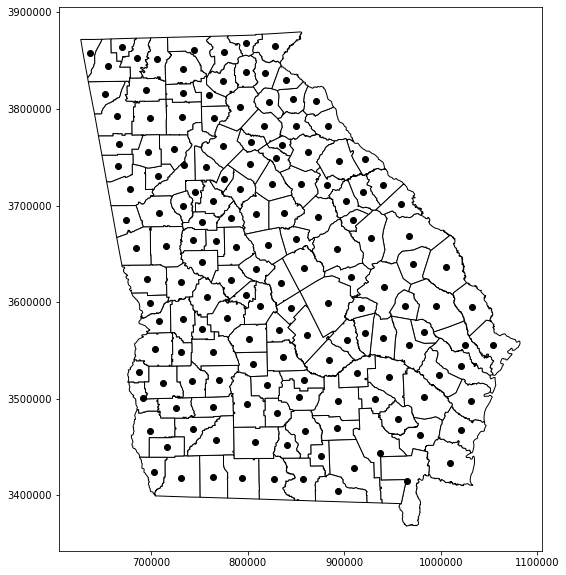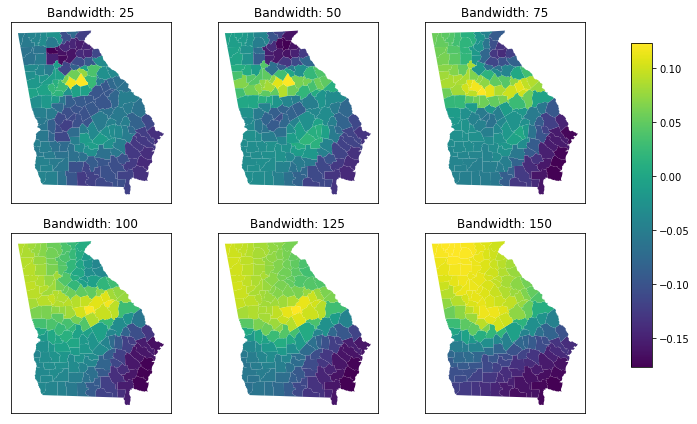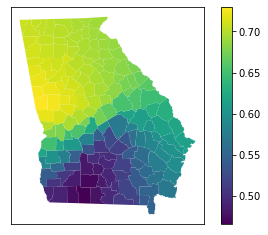# Geographically Weighted Regression Demo Notebook¶

Author: Rebecca Vandewalle rcv3@illinois.edu
Created: 5-19-21

This notebook demonstrates how to preform Geographically Weighted Regression using the MGWR Python package using sample code included in Oshan et al. 2019. MGWR: A Python Implementation of Multiscale Geographically Weighted Regression for Investigating Process Spatial Heterogeneity and Scale. ISPRS Int. J. Geo-Inf. 2019, 8(6), 269; https://doi.org/10.3390/ijgi8060269.

## Install MGWR Python Package¶

The following line and installs the MGWR Python Package if it is not already installed. Documentation for the package can be found here, and documentation for the geographically weighted regression section can be found here.

In :
try:
from mgwr.gwr import GWR
except:
print('Installing MGWR')
! pip install -U mgwr


## Import Required Packages¶

These packages are required to run the sample code.

In :
# code in this cell is from Oshan et al. 2019

import numpy as np
import pandas as pd
import libpysal as ps
from mgwr.gwr import GWR, MGWR
from mgwr.sel_bw import Sel_BW
from mgwr.utils import compare_surfaces, truncate_colormap
import geopandas as gp
import matplotlib.pyplot as plt
import matplotlib as mpl


Here data for counties in Georgia is loaded from an example dataset. The base data is mapped using matplotlib.

In :
# import Georgia dataset and show counties
# code in this cell is from Oshan et al. 2019

fig, ax = plt.subplots(figsize = (10, 10))
georgia.plot(ax=ax, **{'edgecolor': 'black', 'facecolor': 'white'})
georgia.centroid.plot(ax = ax, c = 'black')
plt.show()### Look at data structures¶

In the next cells, the data formats used for the example are illustrated.

In :
# inspect georgia data type

type(georgia)

Out:
geopandas.geodataframe.GeoDataFrame
In :
# inspect base data structure


Out:
AREA PERIMETER G_UTM_ G_UTM_ID Latitude Longitud TotPop90 PctRural PctBach PctEld PctFB PctPov PctBlack X Y AreaKey geometry
0 1.331370e+09 207205.0 132 133 31.75339 -82.28558 15744 75.6 8.2 11.43 0.64 19.9 20.76 941396.6 3521764 13001 POLYGON ((931869.062 3545540.500, 934111.625 3...
1 8.929300e+08 154640.0 157 158 31.29486 -82.87474 6213 100.0 6.4 11.77 1.58 26.0 26.86 895553.0 3471916 13003 POLYGON ((867016.312 3482416.000, 884309.375 3...
2 7.434020e+08 130431.0 148 146 31.55678 -82.45115 9566 61.7 6.6 11.11 0.27 24.1 15.42 930946.4 3502787 13005 POLYGON ((914656.875 3512190.000, 924718.375 3...
3 9.053950e+08 185737.0 158 155 31.33084 -84.45401 3615 100.0 9.4 13.17 0.11 24.8 51.67 745398.6 3474765 13007 POLYGON ((744258.625 3480598.500, 765025.062 3...
4 6.941830e+08 151347.0 76 79 33.07193 -83.25085 39530 42.7 13.3 8.64 1.43 17.5 42.39 849431.3 3665553 13009 POLYGON ((832974.188 3677273.500, 834048.688 3...
In :
# inspect centroids


Out:
0    POINT (946421.511 3522063.064)
1    POINT (892248.432 3469655.794)
2    POINT (931804.516 3499689.285)
3    POINT (743153.933 3468328.184)
4    POINT (850194.951 3664941.794)
dtype: geometry

## Preprocess Data for GWR¶

Data is transformed into the expected format for loading into the GWR model.

In :
# process input data for GWR
# code in this cell is from Oshan et al. 2019

g_y = georgia['PctBach'].values.reshape((-1, 1))
g_X = georgia[['PctFB', 'PctBlack', 'PctRural']].values
u = georgia['X']
v = georgia['Y']
g_coords = list(zip(u, v))


### View format of processed data¶

In :
# inspect data contents

print('g_y:\n', g_y[:5])
print('\ng_X:\n', g_X[:5])
print('\nu:\n', list(u[:5]))
print('\nv:\n', list(v[:5]))
print('\ng_coords:\n', g_coords[:5], "\n")

g_y:
[[ 8.2]
[ 6.4]
[ 6.6]
[ 9.4]
[13.3]]

g_X:
[[  0.64  20.76  75.6 ]
[  1.58  26.86 100.  ]
[  0.27  15.42  61.7 ]
[  0.11  51.67 100.  ]
[  1.43  42.39  42.7 ]]

u:
[941396.6, 895553.0, 930946.4, 745398.6, 849431.3]

v:
[3521764, 3471916, 3502787, 3474765, 3665553]

g_coords:
[(941396.6, 3521764), (895553.0, 3471916), (930946.4, 3502787), (745398.6, 3474765), (849431.3, 3665553)]



## Set GWR Bandwidth¶

Here the model bandwidth is determined computationally.

In :
# select bandwidth computationally
# code in this cell is from Oshan et al. 2019

gwr_selector = Sel_BW(g_coords, g_y, g_X)
gwr_bw = gwr_selector.search()
print(gwr_bw)

117.0


## Fit GWR Model¶

Here the model is constructed and fitted to the input.

In :
# fit the model
# code in this cell is from Oshan et al. 2019

gwr_model = GWR(g_coords, g_y, g_X, gwr_bw)
gwr_results = gwr_model.fit()
print(gwr_results.resid_ss)

1650.8596982770282


## Plot Changes in Bandwidth¶

The model is fitted several times with different bandwidths, and differences are plotted.

In :
# visualize effects of changing bandwidth
# code in this cell is from Oshan et al. 2019

fig, ax = plt.subplots(2, 3, figsize = (10, 6))
bws = (x for x in range(25, 175, 25))
vmins = []
vmaxs = []
for row in range(2):
for col in range(3):
bw = next(bws)
gwr_model = GWR(g_coords, g_y, g_X, bw)
gwr_results = gwr_model.fit()
georgia['rural'] = gwr_results.params[:, -1]
georgia.plot('rural', ax = ax[row, col])
ax[row,col].set_title('Bandwidth: ' + str(bw))
ax[row,col].get_xaxis().set_visible(False)
ax[row,col].get_yaxis().set_visible(False)
vmins.append(georgia['rural'].min())
vmaxs.append(georgia['rural'].max())
sm = plt.cm.ScalarMappable(norm=plt.Normalize(vmin=min(vmins), vmax=max(vmaxs)))
fig.tight_layout()
cax = fig.add_axes([0.92, 0.14, 0.03, 0.75])
sm._A = []
cbar = fig.colorbar(sm, cax=cax)
cbar.ax.tick_params(labelsize=10)
plt.show()## Assess and Plot Model Fit¶

Finally, measures of global and local fit are computed.

In :
# assess global model fit
# code in this cell is from Oshan et al. 2019

gwr_selector = Sel_BW(g_coords, g_y, g_X)
gwr_bw = gwr_selector.search()
gwr_model = GWR(g_coords, g_y, g_X, gwr_bw)
gwr_results = gwr_model.fit()
print(gwr_results.aic)
print(gwr_results.aicc)
print(gwr_results.R2)

848.9154070534352
851.3502927844658
0.6780742669593464

In :
# assess local model fit
# code in this cell is from Oshan et al. 2019

georgia['R2'] = gwr_results.localR2
georgia.plot('R2', legend = True)
ax = plt.gca()
ax.get_xaxis().set_visible(False)
ax.get_yaxis().set_visible(False)
plt.show()In [ ]: